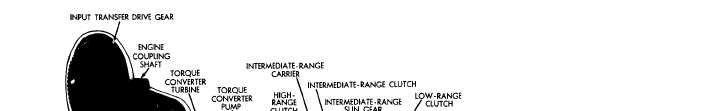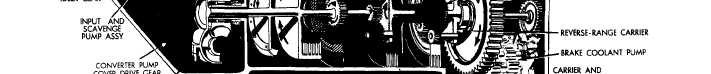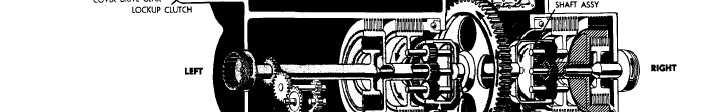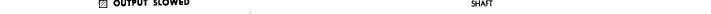Figure   31. Third-gear,   full-right-steer   torque   path,   converter   operation
Custom SearchC H A P    2,   SEC   V T O R Q U E     P A T HS P A R   5   5 55. Figure   31. Third-gear,   full-right-steer   torque   path,   converter   operation TORQUE   PATH - THIRD   GEAR, FULL-RIGHT    STEER,    CONVERTER OPERATION   (fig.   31) .   T o r q u e    i s    t r a n s m i t t e d    t o    t h e    s t e e r planetary   ring   gears   in   the   same   manner   as d e s c r i b e d    i n    p a r .    4 9 a    a n d   b  ,    a b o v e .     F r om t h i s    p o i n t ,    t o    t h e    p o w e r    t r a i n    o u t p u t s ,    t he torque   paths   for   the   right   and   left   sides   are d i f f e r e n t. .   T h e    g e a r e d    s t e e r    m e t h o d    i s    u s e d    f or t h i r d - g e a r    s t e e r .    I n    t h i s    m e t h o d ,    s t e e r i ng r e s u l t s    w h e n    t h e    g e a r e d    s t e e r    c l u t c h    i s    e n- gaged   at   one   side   while   the   drive   clutch   (out- put   clutch)   is   applied   at   the   opposite   side.   The vehicle    steers    toward    the    side    on    which    the geared   steer   clutch   is   engaged. .   In   right   steer,   in   third   gear,   the   right- geared   steer   clutch   is   applied   while   the   right- output   clutch   is   released.   The   drive   clutch   on the   left   side   is   still   engaged   and   continues   to d  r  i  v  e . H y d r a u l i c    a c t i o n ,    c o n t r o l l e d    b y    t h e steer   control   valve,   releases   the   drive   clutch and    applies    the    geared    steer    clutch.    An    op- posite   movement   of   the   steer   control   valve   will release   the   left-output   clutch,   engage   the   left- geared   steer   clutch,   and   result   in   left   steer. .   In   full   steer,   the   applied   geared   steer c l u t c h    l o c k s    t h e    g e a r e d    s t e e r    p l a n e t a r y    s un g e a r     a n d     s l o w s     t h e     g e a r e d     s t e e r     p l a n e t a ry c a r r i e r .    I n    p a r t i a l    s t e e r ,    t h e    g e a r e d    s t e er clutch   is   only   partially   applied   and   slows   the output   to   a   lesser   degree.   The   degree   of   steer is   controlled   by   the   degree   of   movement   of   the s t e e r    c o n t r o l .    R e f e r    t o    T A B U L A T E D    D A T A, par.   7,   for   the   gear   ratios   applicable   to   third g e a r ,    r i g h t    s t e e r . 5 8Integrated Publishing, Inc. - A (SDVOSB) Service Disabled Veteran Owned Small Business